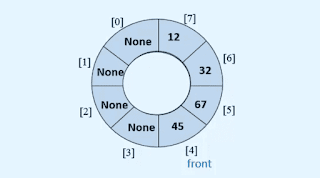A circular queue is a queue in which the front and end parts are connected together and make a circle.## Why we use a Circular queue?

In the queue when we delete the item from the front part then the position from where we deleted the values become vacant or None.and to utilize these positions and to insert new values again we make the circular queue.Note: To make a circular queue we need to give a fixed size to it.

### Enqueue operation

to insert a new value in the queue is called the enqueue operation.

### Dequeue operation

to delete a value from the queue is called the Dequeue operation.

to keep track of the position of the enqueue and dequeue elements we use two variables front and count. initially, we set front and count variables to zero. and then if we enqueue any element in the queue then we increment count variable's value by 1 and when we dequeue an element from the queue we increment the front variable's value by 1.

we use this formula to find the position where we need to insert a new value.

i = ( front + count ) % len( queue )

here the length of the queue is equal to the size of the queue.

and we use this formula to find the position from where we need to delete the element from the queue.

queue[front] = None
front = ( front + 1 ) % len( queue )

here first we need to store the value None into the front position of the queue. and then we use the formula to find the new position of the front.

#### Program to Implement a circular queue using array in a python programming language.

```class EmptyQueueError(Exception):
pass

class Queue:

def __init__(self, default_size=10):
self.items = [None] * default_size
self.front = 0        self.count = 0
def is_empty(self):
return self.count == 0
def size(self):
return self.count

def enqueue(self, item):
if self.count == len(self.items):
self.resize(2 * len(self.items))

i = (self.front + self.count) % len(self.items)
self.items[i] = item
self.count += 1
def dequeue(self):
if self.is_empty():
raise EmptyQueueError("Queue is empty")

x = self.items[self.front]
self.items[self.front] = None        self.front = (self.front + 1) % len(self.items)
self.count -= 1        return x

def peek(self):
if self.is_empty():
raise EmptyQueueError("Queue is empty")
return self.items[self.front]

def display(self):
print(self.items)

def resize(self, newsize):
old_list = self.items
self.items = [None] * newsize
i = self.front
for j in range(self.count):
self.items[j] = old_list[i]
i = (1 + i) % len(old_list)
self.front = 0

###########

if __name__ == '__main__':
qu = Queue(6)

while True:
print("1. Enqueue")
print("2. Dequeue")
print("3. Peek")
print("4. Size")
print("5. Display")
print("6. Quit")

choice = int(input("Enter your choice : "))

if choice == 1:
x = int(input("Enter the element : "))
qu.enqueue(x)
elif choice == 2:
x = qu.dequeue()
print("Element deleted from the queue is : ", x)
elif choice == 3:
print("Element at the front end is ", qu.peek())
elif choice == 4:
print("Size of queue ", qu.size())
elif choice == 5:
qu.display()
elif choice == 6:
break        else:
print("Wrong choice")
print()```